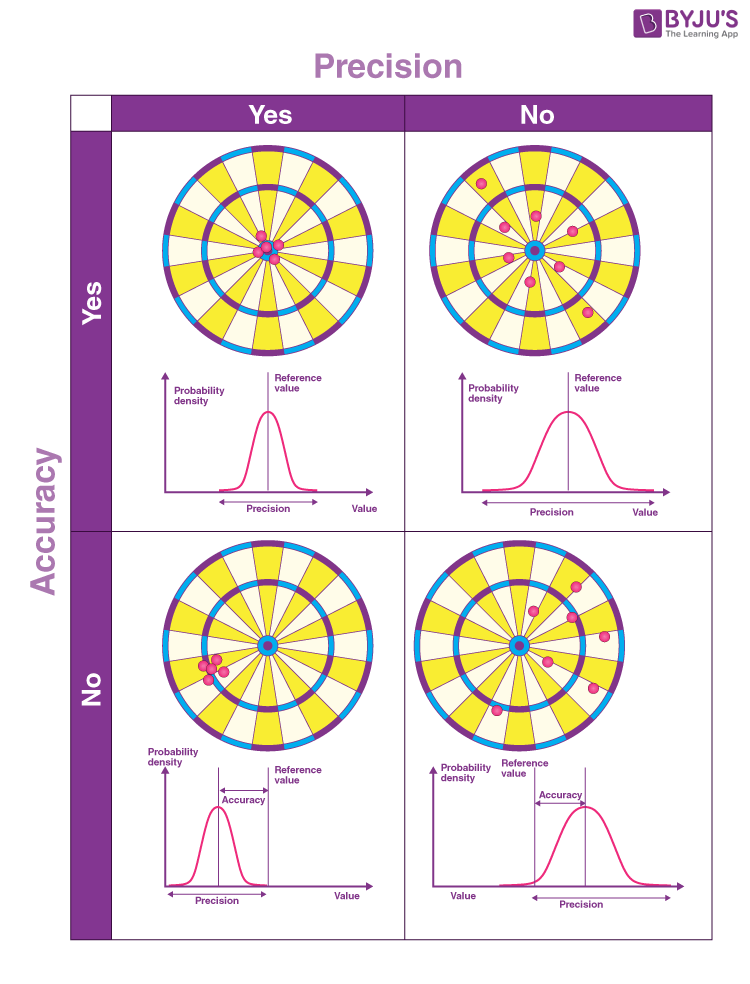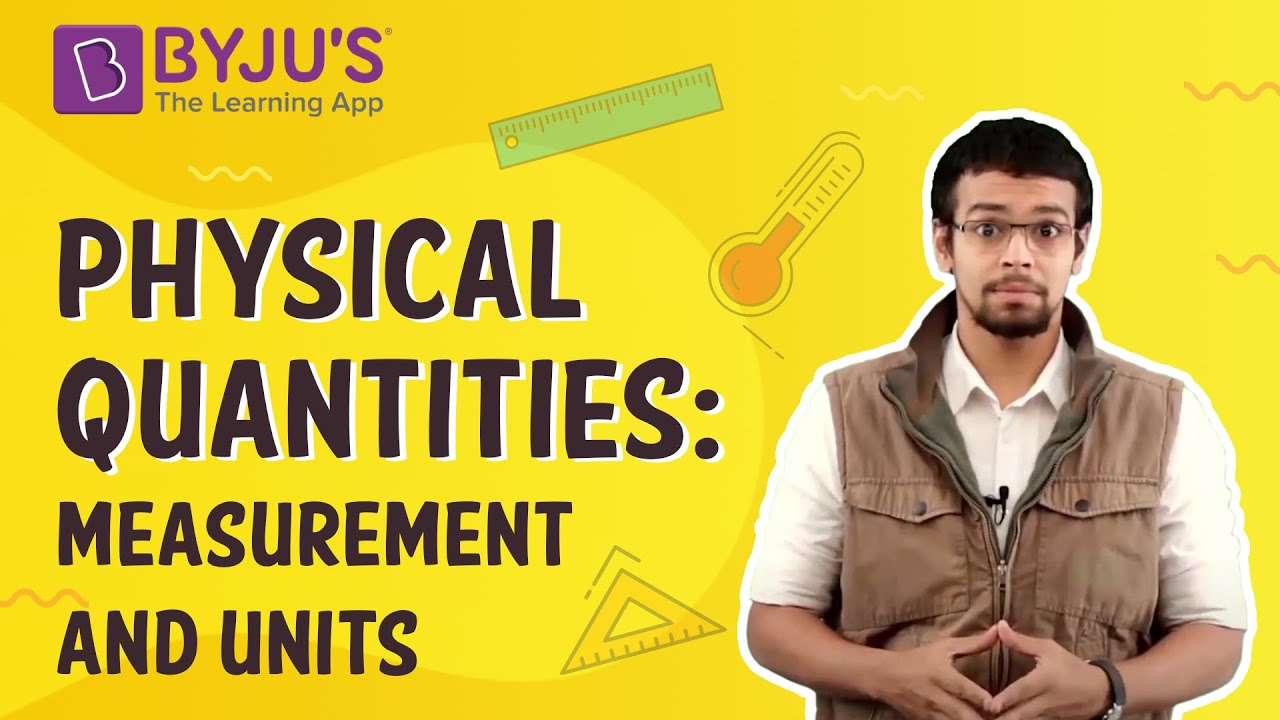# Accuracy and Precision - The Art of Measurement

Measurement is essential for us to understand the external world, and through millions of years of life, we have developed a sense of measurement. Measurements require tools that provide scientists with a quantity. The problem here is that the result of every measurement by any measuring instrument contains some uncertainty. This uncertainty is referred to as an error. Accuracy and precision are two important factors to consider while taking measurements. Both these terms reflect how close a measurement is to a known or accepted value. In this article, let us learn in detail about precision and accuracy.

## Accuracy

The ability of an instrument to measure the accurate value is known as accuracy. In other words, it is the the closeness of the measured value to a standard or true value. Accuracy is obtained by taking small readings. The small reading reduces the error of the calculation. The accuracy of the system is classified into three types as follows:

• Point Accuracy

The accuracy of the instrument only at a particular point on its scale is known as point accuracy. It is important to note that this accuracy does not give any information about the general accuracy of the instrument.

• Accuracy as Percentage of Scale Range

The uniform scale range determines the accuracy of a measurement. This can be better understood with the help of the following example:
Consider a thermometer having the scale range up to 500ºC. The thermometer has an accuracy of ±0.5 percent of scale range i.e. 0.005 x 500 = ± 2.5 ºC. Therefore, the reading will have a maximum error of ± 2.5 ºC.

• Accuracy as Percentage of True Value

Such type of accuracy of the instruments is determined by identifying the measured value regarding their true value. The accuracy of the instruments is neglected up to ±0.5 percent from the true value.

## Precision

The closeness of two or more measurements to each other is known as the precision of a substance. If you weigh a given substance five times and get 3.2 kg each time, then your measurement is very precise but not necessarily accurate. Precision is independent of accuracy. The below examples will tell you about how you can be precise but not accurate and vice versa. Precision is sometimes separated into:

• Repeatability

The variation arising when the conditions are kept identical and repeated measurements are taken during a short time period.

• Reproducibility

The variation arises using the same measurement process among different instruments and operators, and over longer time periods.

### Conclusion

Accuracy is the degree of closeness between a measurement and its true value. Precision is the degree to which repeated measurements under the same conditions show the same results.

Since you are here, you might want to check out the following articles:

## Accuracy and Precision Examples

A good analogy for understanding accuracy and precision is to imagine a football player shooting at the goal. If the player shoots into the goal, he is said to be accurate. A football player who keeps striking the same goalpost is precise but not accurate. Therefore, a football player can be accurate without being precise if he hits the ball all over the place but still scores. A precise player will hit the ball to the same spot repeatedly, irrespective of whether he scores or not. A precise and accurate football player will not only aim at a single spot but also score the goal.The top left image shows the target hit at high precision and accuracy. The top right image shows the target hit at a high accuracy but low precision. The bottom left image shows the target hit at a high precision but low accuracy. The bottom right image shows the target hit at low accuracy and low precision.

### More Examples

• If the weather temperature reads 28 °C outside and it is 28 °C outside, then the measurement is said to be accurate. If the thermometer continuously registers the same temperature for several days, the measurement is also precise.
• If you take the measurement of the mass of a body of 20 kg and you get 17.4,17,17.3 and 17.1, your weighing scale is precise but not very accurate. If your scale gives you values of 19.8, 20.5, 21.0, and 19.6, it is more accurate than the first balance but not very precise.

## Difference between Accuracy and Precision

In the previous few sections having discussed what each term means, let us now look at their differences.

 Accuracy Precision Accuracy refers to the level of agreement between the actual measurement and the absolute measurement. Precision implies the level of variation that lies in the values of several measurements of the same factor. Represents how closely the results agree with the standard value. Represents how closely results agree with one another. Single-factor or measurement are needed. Multiple measurements or factors are needed to comment about precision. It is possible for a measurement to be accurate on occasion as a fluke. For a measurement to be consistently accurate, it should also be precise. Results can be precise without being accurate. Alternatively, the results can be precise and accurate.

## Practice Questions

Q1) The volume of a liquid is 26 mL. A student measures the volume and finds it to be 26.2 mL, 26.1 mL, 25.9 mL, and 26.3 mL in the first, second, third, and fourth trial, respectively. Which of the following statements is true for his measurements?

a. They are neither precise nor accurate.
b. They have poor accuracy.
c. They have good precision.
d. They have poor precision.

Q2) The volume of a liquid is 20.5 mL. Which of the following sets of measurement represents the value with good accuracy?
18.6 mL, 17.8 mL, 19.6 mL, 17.2 mL
19.2 mL, 19.3 mL, 18.8 mL, 18.6 mL
18.9 mL, 19.0 mL, 19.2 mL, 18.8 mL
20.2 mL, 20.5 mL, 20.3 mL, 20.1 mL

Answer: The set 20.2 mL, 20.5 mL, 20.3 mL, 20.1 mL represents the value with good accuracy.

## Frequently Asked Questions – FAQs

Q1

### What is meant by accuracy?

Accuracy refers to the closeness of the measured value to a standard or true value.

Q2

### What is the classification of accuracy of the system?

Accuracy of the system are classified into:

• Point Accuracy
• Accuracy as Percentage of Scale Range
• Accuracy as Percentage of True Value
Q3

True.
Q4

### What is meant by precision?

Precision is the degree to which repeated measurements under the same conditions show the same results.

Q5

### What is meant by error

The difference between the actual value and the measured value is known as error.

Q6

### Write the two factors to be considered while taking measurements?

Two factors to consider while taking measurements are: accuracy and precision.
Q7

### What is point accuracy?

The accuracy of the instrument only at a particular point on its scale is known as point accuracy.
Q8

### Can the results be precise and accurate?

Yes, the results can be precise and accurate.
Q9

### Define reproducibility.

The variation seen in the same measurement process among different operators and instruments over longer time period is referred to as reproducibility.
Q10

### If the player shoots ball into the goal in one shot, he is said to be accurate or precise?

The player is said to be accurate.

## Watch the video to find out what are base measurements